What You Choose Is What You Weigh:

by on December 14th, 2012

As we experience, life is all about laying priorities & importance to the things that affect us. ‘Importance’ in mathematical parlance is referred to as ‘Weight’.

It depends on an individual ‘how much weight one places on making a choice?’

Be it choosing a car or a would-be-spouse, we assign different weights to different attributes. Hope you guys don’t mind if I discuss this aspect using the example of buying a car instead of selecting a would-be-spouse.

Say John wishes to buy a car. He lays importance to 2 attributes—Mileage & Power. He narrows down his choice to two brands of car.

Assuming a scale of 10, Brand A scores 8 on mileage & brand B scores 6. While on a similar scale; Brand A scores 6 on Power & brand B scores 8.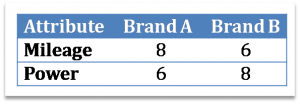It is obvious that if John weighs Mileage & Power equally, then it is difficult for him to choose one car brand over the other. While Brand B scores better in case of Power, Brand A excels in terms of mileage.

Practically speaking, we seldom lay equal importance to all attributes. For people who really care for the weight of their pocket, mileage will be more important than power, while for adventure loving people, it will just be the reverse.

Our friend John is an adventure loving guy, who reckons Power to be relatively more important than Mileage. He lays 60% weight to Power and 40% to Mileage. So here, the scenario will look like this.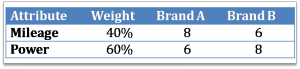Now, in this case, John will clearly prefer Brand B over Brand A since Brand B scores more in terms of Power, which is a more important attribute for him.

But David, another friend of ours, shares a different opinion than John’s. Because he drives a lot on a daily basis, he sets his prority to the brand that gives him value-for-money. He lays 40% weight to Power, & 60% to Mileage. So for him, the scenario will look like this.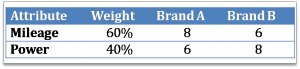It is quite obvious that David will buy brand A rather than brand B.

In this world, there are varied needs of individuals, hence there are multiple brands that co-exist due to different weights assigned by each individual to different needs.

So let’s see how John rates the two brands for cars mathematically. For brand A, contribution of mileage is 40% of 8, equaling to 3.2. Similarly, contribution of power is 60% of 6, equaling to 3.6. So John rates Brand A at 3.2 + 3.6 = 6.8.

For Brand B, contribution of mileage is 60% of 8, equaling to 4.8 and of power is 40% of 6, equaling to 2.4. So John rates brand B as 4.8 + 2.4 = 7.2.

Now, these final rates of both brands of cars are in fact called ‘Weighted Average’.

Well friends, life is not that easy. We frequently come across situations, where a choice may involve multiple attributes with varied importance. But the good news is that even with such plethora of choices, we can easily derive ‘Weighted Average’ with the help of this formula.

Consider a dataset with ‘n’ attributes with their values:

The weighted average would be calculated as follows.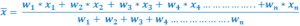Now let’s consider an example with more than 2 attributes. Say another person, Joe, considers 5 attributes to buy a car; Mileage, Power, Cost, Service, & Safety. He assigns relative weights to each attribute as given in the table below (The table also mentions the ratings of 3 brands based on these attributes).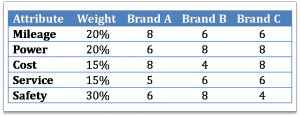For Joe, the final rating for Brand A would be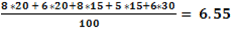Similarly, brand B rates 6.7 and brand C rates 6.1.

Let’s orient our thinking as to how GMAT tests this concept. Let’s discuss one dataset which uses Weighted Average concept in a slightly different way.

‘For its 4-year Bachelor of Engineering (BE) program, the college proposes to the Student Union two-options to determine final score.   In these two approaches, the college wishes to weigh each year marks differently.  Given below are the two suggested options along with sample percentage marks of a student for each year.’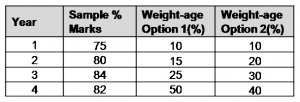For each of the following statements, select Yes’ if statement is true based solely on the information provided in the table; otherwise select ‘No’.

1. If sample marks are taken into account, then Option 1 is more favorable to the Student Union.
2. If sample marks are taken into account, then calculating the final score as the median of marks is more favorable to the Student Union than opting for Option 1.
3. Students who expect to score higher marks in their 4th Year as compared to other 3 years should prefer Option 1 over Option 2.

Understand the dataset

Let’s first understand the data set. When we look at the dataset, we should register the following aspects in our mind.

The data set is about a 4-year college program. As the table shows, marks are allocated each year. The college has suggested two approaches to determine the final score of students. The options vary in terms of the weight assigned to mark each year to calculate the final marks of each student. We have also been provided with 1 set of marks for a student.

Let’s just view a few data points. Weight means importance given to a certain value. In the present example, we can infer that 1st year sample marks carries an importance of 10% only compared to 50% for 4th year marks as per option ‘1’. Note, both columns 3 and 4 individually add up to 100%. This means that for computation of final marks, first year contribution is 7.5 (10% of 75), whereas 4th year contribution is 41 (50% of 82).

Another interesting aspect is that we can expect students who score less in final year to prefer option 2 over option 1. It is because option 2 lays 10 percentage points more weight than option 1 for the marks earned in the 4th year.

Question 1 Statement

If sample marks are taken into account, then Option 1 is more favorable to the Student Union.

Understand the Question

Let’s understand the first question statement. We have to find whether this statement is true or false per the information in the dataset. Now this question statement does not directly provide us with the mathematical relationship. We need to contextualize this statement.

What will the Student Union or students favor more? It will be the option that helps them score higher final marks given their scores in each of the 4 years. So clearly, the answer to this question statement is YES if the final score per option 1 is greater than the final score per option 2.  The mathematical equation is presented below.

Final Score|Option 1 > Final Score|Option 2

And we need to calculate this final score using the sample % marks that we have been provided in the table.

Approach

1. Calculate relative weighted percentage points for option 1 over option 2;

Column 5 = Column 3 – column 4

2. Calculate relative more/less Final Score of option 1 over option

Column 2 & 5 & Apply:3. If calculated value in step 2.a is more than 0, answer is YES, else NO.

Solve-Apply Approach

So now let’s come up with the approach to solve this question.

Let’s understand the question again; we need not calculate the final scores via option 1 & 2. The question asks us ‘Is option 1 final score relatively more than final score via option 2?’

So we simply calculate how much option 1 weight is more or less than option 2 relatively. This will save our precious time of calculating final scores twice i.e. final score for both the options, & then compare their scores.

Let’s put our findings in column 5.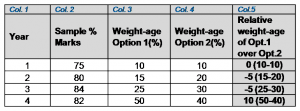Relative more or less  final year score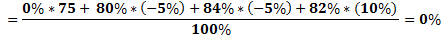We could have excluded year 1 calculation, as the relative weight of option 1 over option 2 is 0. It will not have any impact on final score calculation.

0% means that while calculating final year marks, option 1 does not weigh more or less compared to option 2. Final score via both the options are equal. Hence the answer to this question statement is NO.

Should you wish to look at an interactive audio visual solution of this question, & the subsequent 2 more questions, you may click at ‘START FREE TRIAL’.

We realize that it is the time to address the need of the test takers to attempt variety of IR questions that are true reflection of Official Guide questions. e-GMAT has launched 15+ hours of interactive audio visual content on IR. It encompasses 35 concepts, 120+ original questions, and 2 full length Mock Tests.

We appreciate if you attempt the question, & provide us feedback. We at e-GMAT constantly strive for excellence in learning pedagogy.

Happy Reasoning!

-Shalabh Jain